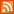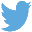Bucaro TecHelpHTTPS Encryption not required because no account numbers orpersonal information is ever requested or accepted by this siteCustom Search# Java Script Code to Factor Numbers

A factor of a number is a whole number that divides into that number with no remainder. Some numbers cannot be evenly divided, examples are 2, 3, 5, and 7. The only factors they have are 1 and themselves. Numbers that can only be divided, with no remainder, by 1 and themselves are called prime numbers. A number that is a factor of a number, and is a prime number, is called a prime factor. If you have the prime factors of a number, you can derive all the other non-prime factors by multiplying several of the prime factors.

Being able to factor numbers is an important skill, one that is necessary to work with equations, fractions and polynomials. In this article, I show you how to use Java Script to factor a number, therefore you will learn a little mathematics and a little programming at the same time.

The method to factor a number is to test if the smallest prime number (2) is a factor of that number. If so, we divide the number by that prime number and test the result again by the smallest prime number, and continue testing until we have extracted all the smallest prime factors. If there is a remainder, we test if the next smallest prime number (3) is a factor of that remainder. We just keep extracting prime factors this way until we end up with no remainder, or a remainder that is a prime number.

So, the first thing we need is a list of all the prime numbers. Unfortunately, there are an infinite number of prime numbers. Fortunately, we don't need all of the prime numbers, we only need the prime numbers that are smaller than the number we want to factor.

It would be an interesting exercise to create a program to calculate a list of the prime numbers, but that has already been done to death, even by super computers. So instead, we'll borrow part of the list found at The First 1,000 Primes

If you want to follow along with this example, copy and paste the example code into a text file. Save the file with a name with the file extension .htm. Then double-click on the file name to open your Web browser and execute the code. shown below is my first Java Script code example, which extracts all the smallest prime number (2) factors from the number 6552.

```<script type="text/javascript">

num = 6552;
var factors = "";

while(num % 2 == 0)
{
factors += "2 ";
num = num/2;
}

alert(factors + " " + num);

</script>
```

The heart of this code is a while loop to which we pass the expression (num % 2 == 0). This means "take the value in the variable num, modulo divide it by 2 (modulo divide works like regular divide except it returns only the remainder), and test if that is zero." As long as that is true (in other words num divides evenly by 2) the while loop will keep executing the code within its brackets.

The code within the while loop brackets first concatenates "2 " to the string named factors, and then divides num by 2 (regular divide). This extracts all the factors of 2 from num. After three factors of 2 are extracted, the remainder is 819, which causes (num % 2 == 0) to return false and program execution drops out of the loop.

 .menubtn { margin-top:10px; margin-left:50px; width:220px; opacity:1; border-style:solid; border-color:#009000; background-color:#66ff66; transition-property: opacity; transition-duration: 1s; transition-timing-function: ease-out; } .menubtn:hover { opacity:1; background-color:#e5ff23; } Menu - More Java Script ProgrammingRSS FeedFollow @Stephen Bucaro[Site User Agreement] [Privacy Policy] [Site map] [Search This Site] [Contact Form]
Copyright©2001-2021 Bucaro TecHelp 13771 N Fountain Hills Blvd Suite 114-248 Fountain Hills, AZ 85268